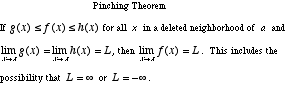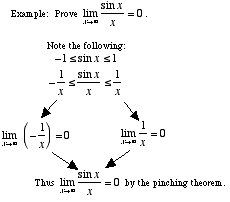# squeeze theorem

Pinching Theorem
Sandwich Theorem
Squeeze Theorem

A theorem which allows the computation of the limit of an expression by trapping the expression between two other expressions which have limits that are easier to compute.